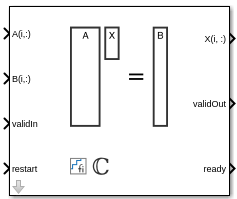# Complex Partial-Systolic Matrix Solve Using QR Decomposition

Compute value of x in Ax = B for complex-valued matrices using QR decomposition

• Library:
• Fixed-Point Designer HDL Support / Matrices and Linear Algebra / Linear System Solvers

•## Description

The Complex Partial-Systolic Matrix Solve Using QR Decomposition block solves the system of linear equations Ax = B using QR decomposition, where A and B are complex-valued matrices. To compute x = A-1, set B to be the identity matrix.

## Ports

### Input

expand all

Rows of matrix A, specified as a vector. A is an m-by-n matrix where m ≥ 2 and mn. If B is single or double, A must be the same data type as B. If A is a fixed-point data type, A must be signed, use binary-point scaling, and have the same word length as B. Slope-bias representation is not supported for fixed-point data types.

Data Types: `single` | `double` | `fixed point`
Complex Number Support: Yes

Rows of matrix B, specified as a vector. B is an m-by-p matrix where m ≥ 2. If A is single or double, B must be the same data type as A. If B is a fixed-point data type, B must be signed, use binary-point scaling, and have the same word length as A. Slope-bias representation is not supported for fixed-point data types.

Data Types: `single` | `double` | `fixed point`

Whether inputs are valid, specified as a Boolean scalar. This control signal indicates when the data from the A(i,:) and B(i,:) input ports are valid. When this value is `1` (`true`) and the ready value is `1` (`true`), the block captures the values at the A(i,:) and B(i,:) input ports. When this value is `0` (`false`), the block ignores the input samples.

After sending a `true` validIn signal, there may be some delay before ready is set to `false`. To ensure all data is processed, you must wait until ready is set to `false` before sending another `true` validIn signal.

Data Types: `Boolean`

Whether to clear internal states, specified as a Boolean scalar. When this value is 1 (`true`), the block stops the current calculation and clears all internal states. When this value is 0 (`false`) and the `validIn` value is 1 (`true`), the block begins a new subframe.

Data Types: `Boolean`

### Output

expand all

Rows of matrix X, returned as a scalar or vector.

Data Types: `single` | `double` | `fixed point`

Whether the output data is valid, returned as a Boolean scalar. This control signal indicates when the data at the output port X(i,:) is valid. When this value is 1 (`true`), the block has successfully computed a row of matrix X. When this value is 0 (`false`), the output data is not valid.

Data Types: `Boolean`

Whether the block is ready, returned as a Boolean scalar. This control signal indicates when the block is ready for new input data. When this value is `1` (`true`) and the validIn value is `1` (`true`), the block accepts input data in the next time step. When this value is `0` (`false`), the block ignores input data in the next time step.

After sending a `true` validIn signal, there may be some delay before ready is set to `false`. To ensure all data is processed, you must wait until ready is set to `false` before sending another `true` validIn signal.

Data Types: `Boolean`

## Parameters

expand all

Number of rows in input matrices A and B, specified as a positive integer-valued scalar.

#### Programmatic Use

 Block Parameter: `m` Type: character vector Values: positive integer-valued scalar Default: `4`

Number of columns in input matrix A, specified as a positive integer-valued scalar.

#### Programmatic Use

 Block Parameter: `n` Type: character vector Values: positive integer-valued scalar Default: `4`

Number of columns in input matrix B, specified as a positive integer-valued scalar.

#### Programmatic Use

 Block Parameter: `p` Type: character vector Values: positive integer-valued scalar Default: `1`

Regularization parameter, specified as a non-negative scalar. Small, positive values of the regularization parameter can improve the conditioning of the problem and reduce the variance of the estimates. While biased, the reduced variance of the estimate often results in a smaller mean squared error when compared to least-squares estimates.

#### Programmatic Use

 Block Parameter: `k` Type: character vector Values: positive integer-valued scalar Default: `0`

Data type of the output matrix X, specified as `fixdt(1,18,14)`, `double`, `single`, `fixdt(1,16,0)`, or as a user-specified data type expression. The type can be specified directly, or expressed as a data type object such as `Simulink.NumericType`.

#### Programmatic Use

 Block Parameter: `OutputType` Type: character vector Values: `'fixdt(1,18,14)'` | `'double'` | `'single'` | `'fixdt(1,16,0)'` | `''` Default: `'fixdt(1,18,14)'`

expand all

## Extended Capabilities

Introduced in R2020b

## SupportGet trial now